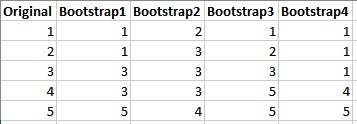### Why should I choose AnalystNotes?

AnalystNotes specializes in helping candidates pass. Period.

##### Subject 6. Resampling

Resampling is a way to reuse data to generate new, hypothetical samples (called resamples) that are representative of an underlying population. Resampling is used when:

• You don't know the underlying distribution for the population,
• Traditional formulas are difficult or impossible to apply,
• As a substitute for traditional methods.

The bootstrap method is a resampling technique used to estimate statistics on a population by sampling a dataset with replacement.

Both bootstrapping and traditional methods use samples to draw inferences about populations. Both can estimate sampling distributions. A primary difference is how they estimate sampling distributions.

Traditional methods use properties of the sample data, the experimental design, a test statistic and need to satisfy the assumptions.

The bootstrap method involves iteratively resampling a dataset with replacement. This method takes the sample data that a study obtains, and then resamples it over and over to create many simulated samples. Each of these simulated samples has its own properties, such as the mean. When you graph the distribution of these means on a histogram, you can observe the sampling distribution of the mean. You don't need to worry about test statistics, formulas, and assumptions.

Example

Suppose a study collects five data points and creates four bootstrap samples:The resampled datasets are the same size as the original dataset and only contain values that exist in the original set. Furthermore, these values can appear more or less frequently in the resampled datasets than in the original dataset. Finally, the resampling process is random and could have created a different set of simulated datasets.

keep in mind that bootstrapping does not create new data. Instead, it treats the original sample as a proxy for the real population and then draws random samples from it. Consequently, the central assumption for bootstrapping is that the original sample accurately represents the actual population. As the sample size increases, bootstrapping converges on the correct sampling distribution under most conditions.

The Jackknife works by sequentially deleting one observation in the data set, then recomputing the desired statistic. It is computationally simpler than bootstrapping. The main application is to reduce bias and evaluate variance for an estimator.

The jackknife requires n repetitions for a sample of n (for example, if you have 10,000 items then you'll have 10,000 repetitions), while the bootstrap requires "B" repetitions. This leads to a choice of B, which isn't always an easy task.

Learning Outcome Statements

i. describe the use of resampling (bootstrap, jackknife) to estimate the sampling distribution of a static;

CFA® 2022 Level I Curriculum, Volume 1, Module 5